Next: 2. Sinusoids Up: course-notes Previous: course-notes   Index

Subsections

# 1. Sounds, Signals, and Recordings

Acoustics is the study of sounds, and for an artist or media researcher, the important things about acoustics might include: how to store and transmit records of sounds; how to use sound to sense things about the environment; how to generate synthetic sounds; or how to achieve a desired sound quality in an environment.

To be able to do things like this you'll need some understanding of how sounds behave in the real world. You'll also need to know something about how human hearing works, and a good bit about how to manipulate representations of sounds using computers.

# 1.1 Using Signals and Recordings to Mediate Sounds

Physically speaking, a sound is time-varying motion of air (or some other medium) with an accompanying change in pressure. Both the motion and the pressure depend on physical location. Knowing the pressure and motion at one point in the air does not inform you what the pressure and motion might be at any other point.

You can visualize sound this way:Sounds can be mediated as signals, which in turn can be mediated as recordings. A signal, or, to be more explicit, an analog signal, is a voltage or current that goes up and down in time analogously to the changing pressure at a fixed point in space. If we ignore for the moment any real-world limitations of accuracy, the analog signal provides an exact description of the time-varying pressure. (Usually an analog signal doesn't reflect the true pressure, but its deviation from the average atmospheric pressure over time, so that it can take both positive and negative values.) Mathematically, a signal may be represented as a real-valued function of time.

Analog signals can be digitized and recorded using a computer or other digital circuitry. A digital recording is just a series of numbers encoded in some digital representation. A single such number is commonly called a sample, although that term is often also used to describe a digital recording (such as you would play using a sampler"), so here I'll try to remember to use the more precise term sample point.

Computer audio workflow usually goes along part or all of the chain of transmissions shown below:The picture starts with a source emitting a real sound in the air. A microphone translates, in real time, the pressure deviation at a single point into an analog signal, encoded as a time-varying voltage. An analog-to-digital converter (ADC) converts the voltages to a digital recording (a series of numbers). These are no longer time-dependent; they may be stored in a file and accessed at a later time.

The rest of the diagram is the first part in reverse: first, a digital-to-analog converter reads the stored numbers and regenerates a time-varying voltage; then a loudspeaker converts the voltages into a sound in the air.

This is the setup for computer-mediated sound manipulation today, used in recording, broadcasting, telephony, music synthesis, sound art, and many other applications. There might be more than one microphone and speaker, and while in digital form the recordings may be stored, combined with other recordings, moved from one place to another, or whatnot. In some situations, only the first or second part of the chain is needed; for instance, a digital keyboard instrument is essentially a computer that generates a recording and converts it into an analog signal.

In no situation is this setup capable of actually reproducing the sound that the bell emits. The microphone only measures the pressure at a single point in space, and the loudspeaker makes a new sound whose pressure variations at points close to the speaker are approximate reconstructions of the measured pressure at the microphone. But (in one way of thinking about it) the microphone does not distinguish among the infinitude of possible directions the sound it picked up was traveling. In theory we would need an infinitude of microphones to allow us to resolve that infinite number of possibly independently time-varying signals.

One other remark: although the recording in the middle of the diagram has no dependence on time, it is still possible to make the whole chain appear as if it is operating in real time, by quickly passing each arriving sample point (after processing it as desired) on to the DAC - perhaps 1/100 of a second or so after it is received from the ADC. That is how real-time audio processing software works.

# 1.2 Frequently Used Signals: Sinusoids and Noise

A sinusoid is a signal that changes sinusoidally in time, or its recording. As a signal (an analog function of time) it takes the form:Here, the variable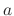is the sinusoid's peak amplitude, in other words, the amplitude (bigness") of the signal at its peak. The variableis the frequency in cycles per unit time. (If time is measured in seconds, then the frequencyis measured in cycles per second, also known as Hertz). The variable(Greek letter phi) is the initial phase; the subscript 0 is there to indicate taht we're talking about the phase at time zero, because we also use the word phase to mean the time-varying phase, equal to.

A sinusoid may be graphed like this (here the initial phase is zero and so isn't shown in the equation):This signal cycles about 2.2 times in the 0.001 seconds shown; from this we can estimate that its frequency is about 2.2 cycles per 0.001 second, or, equivalently, 2200 Hertz.

The signal is periodic, i.e., it repeats the same thing over and over, potentially forever. The period is the number of seconds per cycle, and so it is the reciprocal of the frequency.

As a digital recording, the sinusoid we're looking at might be graphed like this:Instead of a continuous function of time, we see a bar graph with 50 elements. (Alternatively, we could have printed out a list of 50 numerical values). There are 50 sample points per millisecond, or, equivalently, 50,000 sample points per second. We say that the recording has a sample rate of 50,000 samples per second, or to abuse language slightly, 50,000 Hz.

For either a recording or an analog signal, the frequencyand the periodare related by: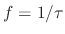or, equivalently,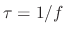. The period is in time units and the frequency in cycles per time unit. In the case of a recording, one might specify time in either seconds or samples. If the sample rate is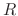samples per second, we may convert frequency or period from one to the other. FOr example, the sinusoid above has a period of about 23 samples (you can count them). To learn the period in seconds, write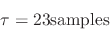and similarly the frequency isHere we can read a unit like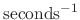equivalently as per second" or, to be more explicit, cycles per second" as we have been doing.

Here, for the record, is what a siuusoid might soind like:

SOUND EXAMPLE: a sinusoid, amplitude 0.1; frequency 1000 cycles per second (Hertz); 5 second duration.

The tone has an audible pitch, which is determined by its frequency. So the parameters(the amplitude) and(the frequency) correspond to audible characteristics of the sinusoid. Under normal conditions you won't hear the initial phase; indeed, if you tune into the sinusoid at some later point in time there will be a different initial phase but it's the same sinusoid with the same sound.

One other elementary signal type recurs throughout any study of acoustics, called white noise. As a recorded signal this is easy to describe: every sample point is a random number betweenand, where, again,denotes the amplitude. (To be more pedantic, this is called uniform white noise to distinguish it from white noise whose sample points are chosen according to a Gaussian or other probability distribution; we won't worry about that here.)

SOUND EXAMPLE: uniform white noise, amplitude 0.1, 5 second duration.

Most people would not say that white noise has an audible pitch, and indeed it has no periodicity. White noise is also different from a sinusoid in that it is not deterministic; it is the result of a random process and if someone else generates a recording of white noise it most likely won't be equal to yours, although it should sound the same. So for instance if I added a sinusoid to a recording of Beethoven's fifth symphony, and if you know its frequency, amplitude, and initial phase, you could subtract the sinusoid back out and recover the original recording; but if I added white noise you wouldn't be able to subtract it out unless I somehow sent you the particular recording of noise I had used.

# 1.3 Units of Pitch and Amplitude

Pitch is often described using logarithmic units (called octaves), for an exceedingly good reason: over the entire range of audible pitches, changing the pitch of a sound by an octave has a very uniform effect on the perceived pitch. Amplitude is also very often described in logarithmic units, called decibels, not because they are the best unit of loudness (that would be sones, to be discussed later) but rather because in sound engineering signals are often put through a series of operations that act multiplicatively on their amplitudes, and in such a situation it is convenient to deal in the logarithms of the amplitude changes so that we can add them instead of having to multiply. (Also, the range of "reasonable" amplitudes between just-audible and dangerously loud can reach a ratio of 100,000; one is immediately tempted to talk in logarithms just to be able to make reasonable graphs.)

Before we go any further I'll risk insulting your intelligence by reviewing logarithms, with musical pitch as the driving example. Choose, for the moment, a reference frequency equal to 440 Hz. We can raise it by octaves by successively doubling it, and lower it by halving it:

FREQUENCY        ...    55   110   220   440   880  1760  3520   ...
RATIO to 440           1/8   1/4   1/2     1     2     4     8
OCTAVES                 -3    -2    -1     0     1     2     3


So if R is the ratio and I is the interval in octaves between 440 Hz. and our frequency, the three are related asor, solving for the interval,As we'll see in Chapter 4 (pitch and musical scales), it is customary in Western musical practice to use a different scale of pitches, measuring them in so-called half steps, defined as one twelfth of an octave:The choice of the reference pitch, 440 Hz, was arbitrary (although that particular frequency is often used as a reference.) If, for instance, we decided to use 220 Hz. as a reference our scale would then look like this:

FREQUENCY        ...    55   110   220   440   880  1760  3520   ...
RATIO to 440           1/4   1/2     1     2     4     8    16
OCTAVES                 -2    -1     0     1     2     3     4
HALF STEPS             -24   -12     0    12    24    36    48


The logarithmic scale of amplitude works similarly. We start by choosing a reference in the appropriate units, which could be, for example, one (for a sound recording), or one volt (for an analog electrical signal) or 0.00002 newtons per square meter (for a pressure deviation in air). Then we can measure the amplitude of any other signal, compared to the reference one, in decibels by an artificial construction that parallels the more natural way we dealt with pitch above. Taking the reference to be one with no units, the decibel scale is set up as shown:

AMPLITUDE           0.01   0.1     1    10   100  1000
DECADES               -2    -1     0     1     2     3
DECIBELS             -40   -20     0    20    40    60


In equations, the relative level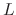is related to the amplitudeby:Here we're explicitly dividing by one, the reference amplitude; if you use a different reference amplitude you should replace the 1" by that reference, the same way you did for the reference frequency, 440, in the calculation of pitch. To make the definitions as general as possible, it is customary to give the reference frequency and amplitudes names--and--to generalize the definitions of relative pitch and level as:As with pitch, there are conventional choices for reference amplitudes, particularly for describing physical sounds, which we'll get to in chapter 6.

# 1.4 Word Size and Sample Rate of Recordings

Since the process of recording is essentially transcribing a continuous, real-valued function of time into a finite-sized array of digits, there is naturally a question of how much precision we will need in order to faithfully reproduce the analog signal we are recording. This has two aspects: first, what should be the precision, the number of binary digits we use to represent each individual sample point? And second, since we can only store a finite number of sample points per second, how many will be enough?

Precision is easily enough understood and decided upon. At stake here is a real number (with a range, for instance, of one volt) being transcribed as a binary number. The average error of the transcription is on the order of the least significant bit. If there are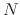bits, this istimes smaller than the range of possible values the-bit number can take. So the error, expressed in decibels with one volt as the reference amplitude, is: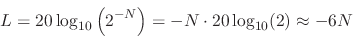In other words, we get 6 decibels of precision for each additional bit we use to encode the sample points. Put another way, the signal-to-noise ratio (often abbreviated as SNR) is.

How much is enough? Well, for day-to-day work, 16 bits (for a SNR of 96 dB) should do it. For exacting situations or those in which you might have some a priori uncertainty as to the level of signal you are dealing with in the first place, it is often desirable to increase the precision further. In professional recording situations it is customary to use 24 bits for an SNR of 144 dB.

The question of sample rate is somewhat trickier. At first consideration one might suppose that a sample rate of 20 kHz should be adequate to represent any signal whose frequencies are limited to 20 kHz (usually considered the upper limit of human hearing). But this doesn't work. Suppose for simplicity that our sample rate is one (that is, one sample for each unit of time), and suppose we want to record a sinusoid whose frequency is also one. Well, we'll sample the sinusoid at time points 0, 1, 2, etc., and... the instantaneous voltages at those time points will all turn out to be equal! We won't get any meaningful recording at all.

The picture below shows this effect in a slightly more general way: here again we set the sample rate to one, and consider the effects of sampling a sinusoid at frequencies of 3/8, 5/8, and 11/8: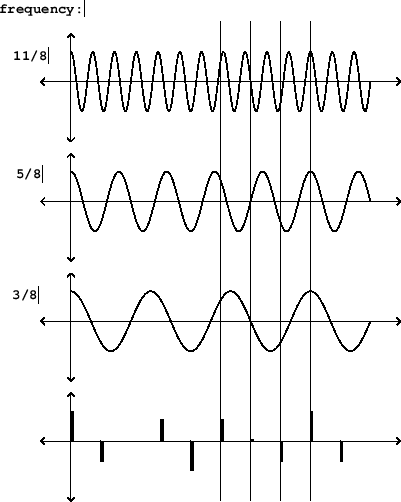Ouch: we get exactly the same recording from the three different sinusoids. This is an example of the phenomenon known as foldover. In general, any sinusoid whose frequency exceeds 1/2 the sample rate (that's called the Nyquist frequency) is exactly equal to another, lower frequency one.

So to represent sinusoids up to a frequency of 20 kHz, we need a sample rate of at least twice that. Because of various engineering considerations we will need an additional margin. The "standard" sample rates in widest use in digital audio are 44100 Hz. (44.1 kHz), called the consumer" or CD" sample rate, or 48 kHz, called the professional" one (although for various reasons people often record at higher rates still).

It is easy to come by a signal that is out of the range of human hearing, either electronically or physically; and it is also easy to write computer algorithms that generate frequencies above the Nyquist frequency as digital recordings. But once such a signal is recorded, standard playback hardware (DACs) will re-create them as the equivalent sinusoid, if any, that is within the range of human hearing, according to the following chart (which uses 48 kHz as the sample rate):The phenomenon of synthesizing or recording one frequency and hearing another because of this ambiguity of frequencies in digital recordings is called foldover.

Recording sound at high quality can require a fair amount of memory; a six-channel, 48 kHz, 24-bit, one-hour recording would require over 3 gigabytes of storage, which might be inconvenient to store on one's computer and worse than inconvenient to share on a website, for example. For this reason much research has been done on data compression for audio recordings, and formats are now available that do an excellent job of reducing the size of a digital recording without changing the audible contents very much. These techniques would take many of pages of equations to describe, and anyway most people don't seem to want to know how they work.

# 1.5 Fundamental Operations: Amplification, Mixing, and Delay

Once a sound is in the form of an analog signals or a digital recording, we can perform a variety of operations on it, three of which con be considered the most fundamental.

Amplification is the process of multiplying the signal or recording by a constant. Ifis a signal, the result is another function of time,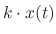. Ifis nonzero, and if (by any measure) the level of the original signal is, the result will have a level equal to:Ifis negative, the signal will also have been inverted. The constantis called the gain. The change in level,, is called the gain in dB".

Mixing is the process of adding two or more signals. (The term is often enlarged to mean amplifying them by various gains and then summing".) The result is usually louder than any of the signals to be summed, but not necessarily.

Delay refers to the process whereby a signal is replaced with an earlier copy of itself. Again usingto denote the original signal, choose a positive time value(Greek tau). The delayed signal is then. Note that we caon't apply a negative delay to a signal in time; that would amount to predicting the future (just tune into tomorrow's news).

The first two of these operations are applied to digital recordings in the same way as to real-time analog signals, but the last one, delay, is slighty different. If we have stored a recording of a sound, we can delay it by simply copying the numbers in the recording to the right or left (or up or down if you're imagining them vertically). The delay" can be negative or positive. But note that, if you are trying to create the impression of real-time processing by continuously outputting the sample points soon after they arrive, you won't be able to shift them forward in time for the same reason you can't do that with an analog signal.

# Exercises and Project

1. A recorded sinusoid has a sample rate of 48 kHz and a frequency of 440 Hz. What is its period in samples?

2. If a 1 volt amplitude signal is raised by 6 decibels, what's the resulting voltage?

3. What frequency is 1/2 octave above 440 Hz.?

4. If you record a signal with a word length of 8 bits, what is the theoretical signal-to-noise ratio?

5. If you generate a sinusoid of frequency 40 Khz, but only sample your sinusoid at a rate of 44.1 kHz, what frequency will you hear when you play it?

6. How many octaves are there in the human hearing range (between 20 and 20,000 Hz.)?

Project: Why you shouldn't trust your computer's speaker. In this project, you will determine your threshold of hearing as a function of frequency: that is, for each frequency, the minimum relative level at which you can hear whether a sinusoid is present or not. This is a generalization of you hearing range: outside your hearing range the threshold is infinite (no matter how loud you play the sound you won't hear it), but you would expect your ears to be somewhat less sensitive to the extremes than the middle as well.

This has been measured for "typical" young humans with increasing reliability and accuracy ever since a set of pioneering experiments in the 1930s by Fletcher and Munson; here is a good up-to-date article. The bottom curve in the graph is the "normal" threshold of hearing.

To do this yourself, get Pd and this patch library, following directions until you have verified that you can make sinusoid" and output" objects in a Pd document. All the patch you have to make is to connect a sinusoid" to an "output".

Then, setting the sinusoid" frequency to 1000, try one level (in dB) after another in the output" object until you find a level at which you don't think you hear the difference when you toggle the sound on and off (using the toggle in the output" object). This will be a crude process and you are unlikely to be sure to plus or minus 5 dB or so where the actual threshold lies. (It might also change with practice, or if you change you sitting posture, etc.) Use your computer speaker (if it has one), or headphones.

If, at 1000 Hz, you get a value above about 50, you might not be able to follow the curve as it rises at other frequencies; if so, try to turn your computer volume up so that you can turn the output" control down lower.

Once you have this working at 1000, try other frequencies at octaves from it (going up: 2000, 4000, 8000, 16000; and going down: 500. 250. 125. 63. 31. 16) finding anew the threshold at each frequency and plotting it. If you can't hear it at all the answer is "infinite".

Graph this as best you can, and also write down whether you used the speaker on your laptop, or something else (headphones, stereo speakers, wires on the tongue, ...) How does the curve you got differ from the one in the link, and why might that be?Next: 2. Sinusoids Up: course-notes Previous: course-notes   Index
msp 2014-11-24